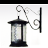# How to evaluate empty field?

785
4
01-16-2012 10:15 PMNew Contributor
Hi guys,

I have a situation with ModelBuilder... I need to know if my field (street) has value or empty from selected features.

I have input parameter for the field (street), if the field is empty, I have to input (entry) a street name. Then calculate field.

IF the field has value, if possible, make the street parameter entry disabled (even though I have set its type to optional in the model properties).

Thanks
jon
Tags (3)
1 Solution

Accepted SolutionsbyMVP Esteemed Contributor
If you want to have the tool simply not tromp any already-set values, within your model you could run a select features by attribute on the input table view (for blank or null values) before running calculate field.

Input table -> Select Layer By attribute -> (table)  -> Calculate Field -> (table) -> Select Layer By Attribute (clear selection)

This would be less complicated than setting up a script tool and doing parameter validation as I suggested.

Another thing you may want to try in your model is not do anything if zero or more than a certain amount of rows are selected, so you don't accidentally calculate all rows. This would be done using the Get Count tool, sending its output to calculate value with an expression like "%Count% > 0 or %Count% < 100", and using the output as a precondition:

Input table -> Select Layer By attribute -> (table) -> Get Count -> (count) -> Calculate value -> (output) -> [precondition] -> Calculate Field -> (table) -> Select Layer By Attribute (clear selection)
4 RepliesbyMVP Esteemed Contributor
What you're trying to do is is something called parameter validation -- and you can only do this with a script tool.

You could write a very short Python script tool that just imports arcpy and calls your model, so you can set up validation on its arguments.New Contributor
What you're trying to do is is something called parameter validation -- and you can only do this with a script tool.

You could write a very short Python script tool that just imports arcpy and calls your model, so you can set up validation on its arguments.

The main problem is how may I determine if field 'Street' is empty (or null) before making an entry of street name, this is from a selected features.

[ATTACH=CONFIG]11239[/ATTACH]byMVP Esteemed Contributor
If you want to have the tool simply not tromp any already-set values, within your model you could run a select features by attribute on the input table view (for blank or null values) before running calculate field.

Input table -> Select Layer By attribute -> (table)  -> Calculate Field -> (table) -> Select Layer By Attribute (clear selection)

This would be less complicated than setting up a script tool and doing parameter validation as I suggested.

Another thing you may want to try in your model is not do anything if zero or more than a certain amount of rows are selected, so you don't accidentally calculate all rows. This would be done using the Get Count tool, sending its output to calculate value with an expression like "%Count% > 0 or %Count% < 100", and using the output as a precondition:

Input table -> Select Layer By attribute -> (table) -> Get Count -> (count) -> Calculate value -> (output) -> [precondition] -> Calculate Field -> (table) -> Select Layer By Attribute (clear selection)New Contributor
If you want to have the tool simply not tromp any already-set values, within your model you could run a select features by attribute on the input table view (for blank or null values) before running calculate field.

Input table -> Select Layer By attribute -> (table)  -> Calculate Field -> (table) -> Select Layer By Attribute (clear selection)

This would be less complicated than setting up a script tool and doing parameter validation as I suggested.

Another thing you may want to try in your model is not do anything if zero or more than a certain amount of rows are selected, so you don't accidentally calculate all rows. This would be done using the Get Count tool, sending its output to calculate value with an expression like "%Count% > 0 or %Count% < 100", and using the output as a precondition:

Input table -> Select Layer By attribute -> (table) -> Get Count -> (count) -> Calculate value -> (output) -> [precondition] -> Calculate Field -> (table) -> Select Layer By Attribute (clear selection)

Thanks sir, you have given me a very good idea and I have managed to apply it to my ModelBuilder... see attached pic... this modelbuilder get-street-field-value, now I know if street field has value or null... this work for me even better! Thanks again... ^_^

[ATTACH=CONFIG]11287[/ATTACH]